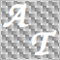• Información general
• Comentarios (2)
• Discusión (1)

# MA With Key Charts

This version contains two components:

• HM Average
• Timeframes in one chart displayed using numeric keys

HM Average is developed by Alan Hull. It is an extremely fast and smooth moving average that almost eliminates lag altogether and manages to improve smoothing at the same time.

To calculate it, first you have to calculate a difference between two LWMA of periods and then calculate another LWMA from this difference but with a period of square root.

### Parameters

• Buffers = 2
• Period = 13
• Shift = 0
• Price = 1

• Buy = closing bar finished above the line
• Sell = closing bar finished below the line
• Exit = opposite of above mentioned Buy and Sell Strategy
• Chart = as I said before, numeric keys will make user's work easy to define a suitable time but I recommend high timeframes (1 Hour, 4 Hours, Daily)

### Timeframe Numeric Keys

• 1 = 1 Minute
• 2 = 5 Minutes
• 3 = 15 Minutes
• 4 = 30 Minutes
• 5 = 1 Hour
• 6 = 4 Hours
• 7 = Daily
• 8 = Weekly
• 9 = Monthly

I hope users will enjoy this indicator.7769
2018.12.07 17:42

thanks for share21363
2016.08.31 09:19

El usuario no ha dejado ningún comentario para su valoración